# Sketch

Next: , Previous: (dir), Up: (dir)

## Sketch

Copyright © 2005, 2006, 2007, 2008 Eugene K. Ressler.

This manual is for sketch, version 0.2 (build 131), Saturday, August 09, 2008, a program that converts descriptions of simple three-dimensional scenes into static drawings. This version generates PSTricks or PGF/TikZ code suitable for use with the TeX document processing system.

Sketch is free software; you can redistribute it and/or modify it under the terms of the GNU General Public License as published by the Free Software Foundation; either version 3, or (at your option) any later version.

Sketch is distributed in the hope that it will be useful, but WITHOUT ANY WARRANTY; without even the implied warranty of MERCHANTABILITY or FITNESS FOR A PARTICULAR PURPOSE. See the GNU General Public License for more details.

You should have received a copy of the GNU General Public License along with sketch; see the file COPYING.txt. If not, see http://www.gnu.org/copyleft.

--- The Detailed Node Listing ---

About sketch

Introduction by example

Swept objects

Input language

Basics

Literals

Arithmetic expressions

Options

Point lists

Drawables

Sweeps

Definitions

Global environment

Building a drawing

Caveats

Hidden surface removal and polygon splitting

Next: , Previous: Top, Up: Top

## 1 About sketch

Sketch is a small, simple system for producing line drawings of two- or three-dimensional objects and scenes. It began as a way to make illustrations for a textbook after we could find no suitable tool for this purpose. Existing scene processors emphasized GUIs and/or photo-realism, both un-useful to us. We wanted to produce finely wrought, mathematically-based illustrations with no extraneous detail.

Sketch accepts a tiny scene description language and generates PSTricks or TikZ/PGF code for LaTeX. The sketch language is similar to PSTricks, making it easy to learn for current PSTricks users. See www.pstricks.de for information on PSTricks. TikZ/PGF are also very similar except for details of syntax. See http://sourceforge.net/projects/pgf. One can easily lay raw PSTricks or TikZ/PGF output over, in, or under sketch drawings, providing the full power of LaTeX text and mathematics formatting in a three-dimensional setting.

Next: , Previous: About sketch, Up: About sketch

### 1.1 Reporting bugs and recommending improvements.

Send bug reports and suggestions to sketch@frontiernet.net. We will try to respond, but can't promise. In any event, don't be offended if a reply is not forthcoming. We're just busy and will get to your suggestion eventually.

For bugs, attach a sketch input file that causes the bad behavior. Embed comments that explain what to look for in the behavior of sketch or its output.

A recommendation for improvement from one unknown person counts as one vote. We use overall vote tallies to decide what to do next as resources permit. We reserve the right to a assign any number of votes to suggestions from people who have been helpful and supportive in the past.

Previous: Reporting bugs, Up: About sketch

### 1.2 Contributions

If you intend to implement an enhancement of your own, that's terrific! Consider collaborating with us first to see if we're already working on your idea or if we can use your work in the official release.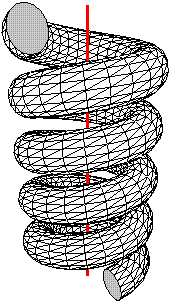Next: , Previous: About sketch, Up: Top

## 2 Introduction by example

The sketch input language will seem familiar to users of the PSTricks package for LaTeX. The following program draws a triangular polygon pierced by a line.

  polygon(0,0,1)(1,0,0)(0,1,0)
line(-1,-1,-1)(2,2,2)

The coordinate system is a standard right-handed Cartesian one.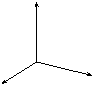Next: , Previous: Introduction by example, Up: Introduction by example

### 2.1 Hello world

The sketch program above is nearly the simplest one possible, the equivalent of a “hello world” program you might find at the start of a programming language text. If it is saved in the file simple.sk, then the command

  sketch simple.sk -o simple.tex

creates a file simple.tex containing PSTricks commands to draw these objects on paper. The contents of simple.tex look like this.
  \begin{pspicture}(-1,-1)(2,2)
\pstVerb{1 setlinejoin}
\psline(-1,-1)(.333,.333)
\pspolygon[fillstyle=solid,fillcolor=white](0,0)(1,0)(0,1)
\psline(.333,.333)(2,2)
\end{pspicture}

The hidden surface algorithm of sketch has split the line into two pieces and ordered the three resulting objects so that the correct portion of the line is hidden.

If you've noticed that the projection we are using seems equivalent to erasing the z-coordinate of the three-dimensional input points, pat yourself on the back. You are correct. This is called a parallel projection. The z-coordinate axis is pointing straight out of the paper at us, while the x- and y-axes point to the right and up as usual.

The resulting picture file can be included in a LaTeX document with \input{simple}. Alternately, adding the command line option -T1 causes the pspicture to be wrapped in a short but complete document, ready to run though LaTeX. In a finished, typeset document, the picture looks like this. (The axes have been added in light gray.)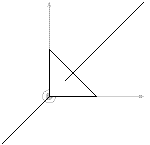It is important to know that only the “outside” of a polygon is normally drawn. The outside is where the vertices given in the polygon command appear in counter-clockwise order. Thus, if the command above had been

  polygon(0,1,0)(1,0,0)(0,0,1)

the polygon would not appear in the picture at all. It would have been culled from the scene. This culling behavior may seem strange, but stay tuned.

Next: , Previous: Hello world, Up: Introduction by example

### 2.2 Options

Many PSTricks and TikZ/PGF options work just fine in sketch. If generating PSTricks, the code

  polygon[fillcolor=lightgray,linewidth=3pt](0,0,1)(1,0,0)(0,1,0)
line[linestyle=dotted](-1,-1,-1)(2,2,2)

produces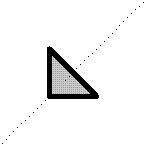To produce TikZ/PGF, the corresponding code is

  polygon[fill=lightgray,line width=3pt](0,0,1)(1,0,0)(0,1,0)
line[style=dotted](-1,-1,-1)(2,2,2)
global { language tikz }

The final global instructs sketch to produce TikZ/PGF code as output rather than the default, PSTricks. Note that polygon fill color and line style options both conform to TikZ syntax rules. The remaining examples of this manual are in PSTricks style.

Next: , Previous: Drawing options, Up: Introduction by example

### 2.3 Drawing a solid

Let's try something more exciting. Sketch has no notion of a solid, but polygonal faces can be used to represent the boundary of a solid. To the previous example, let's add three more triangular polygons to make the faces of an irregular tetrahedron.

  % vertices of the tetrahedron
def p1 (0,0,1) def p2 (1,0,0)
def p3 (0,1,0) def p4 (-.3,-.5,-.8)

% faces of the tetrahedron.
polygon(p1)(p2)(p3) % original front polygon
polygon(p1)(p4)(p2) % bottom
polygon(p1)(p3)(p4) % left
polygon(p3)(p2)(p4) % rear

% line to pierce the tetrahedron
line[linecolor=red](-1,-1,-1)(2,2,2)

This example uses definitions, which begin with def. These define or give names to points, which are then available as references by enclosing the names in parentheses, e.g. (foo). The parentheses denote that the names refer to points; they are required. There can be no white space between them and the name.

As you can see, comments start with % as in TeX and extend to the end of the line (though # will work as well). White space, including spaces, tabs and blank lines, has no effect in the sketch language.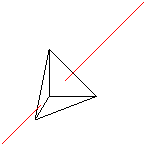If we look inside the TeX file produced by sketch, there will be only three polygons. The fourth has been culled because it is a “back face” of the tetrahedron, invisible to our view. It is unnecessary, and so it is removed.

In some drawings, polygons act as zero-thickness solid surfaces with both sides visible rather than as the faces of solid objects, where back faces can be culled. For zero-thickness solids, culling is a problem. One solution is to use a pair of sketch polygons for each zero-thickness face, identical except with opposite vertex orders. This is unwieldy and expensive. A better way is to set the sketch internal option cull to false in the usual PSTricks manner.

  polygon[cull=false](p1)(p2)(p3)

The following shows the same helix shape drawn first with cull=true (the default) and then cull=false.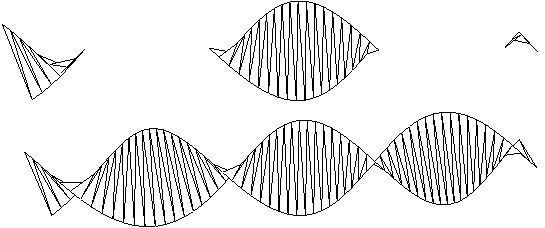We'll soon see how to produce these helixes with a few lines of sketch language code.

It may be tempting to turn culling off gratuitously so that vertex order can be ignored. This is not a good idea because output file size and TeX and Postscript processing time both depend on the number of output polygons. Culling usually improves performance by a factor of two. On the other hand, globally setting cull=false is reasonable while debugging. See Global options and Limits on error detection.

Next: , Previous: Drawing a solid, Up: Introduction by example

### 2.4 Special objects

We can add labels to a drawing by using special objects, which provide a way to embed raw LaTeX and PSTricks code. Adding this to the tetrahedron does the trick.

  special |\footnotesize
\uput{2pt}[ur]#1{$P1$}
\uput[r]#2{$P2$}
\uput[u]#3{$P3$}
\uput[d]#4{$P4$}|
(p1)(p2)(p3)(p4)

Here is the result.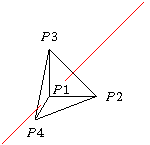There are several details to note here. First, the quoting convention for the raw code is similar to the LaTeX \verb command. The first non-white space character following special is understood to be the quote character, in this case |. The raw text continues until this character recurs.

Second, the argument references #1, #2, #3, and #4 refer to points in the list that follow. This is similar to TeX macro syntax. The transformed and two-dimensional projections of these three-dimensional points are substituted in the final output. An argument reference of the form #1-2 is replaced with the angle in degrees of the two-dimensional vector that connects the projections of the two respective argument points, here #1 and #2. The substituted angle is enclosed in curly braces { }

By default, special objects are printed last, overlaying all other objects in the scene. If you specify the internal option lay=in, the hidden surface algorithm considers the entire special object to be the first point (#1) in the argument list. If that point is behind (of smaller z-component than) any drawable, then the entire special object is drawn before that drawable, so the drawable obscures parts of the special object that overlaps it. In our example, p1 is the front-most point in the scene (has the largest z-component), so adding lay=in has no effect.

With option lay=under, a special is drawn before, hence appears under any of the objects handled by the hidden surface algorithm. This is how the light gray axes were added to the “hello world” example Hello world.

Special objects are powerful, with many possible uses.

Next: , Previous: Special objects, Up: Introduction by example

### 2.5 Transforms

Now let's add a second copy of the pierced tetrahedron. We'll rotate the copy 90 degrees about the x-axis with the origin as center of rotation so we can see the back, then translate it to the right—in the positive x-direction—so it doesn't collide with the original. To help us see what's going on, make the back side gray.

  def pierced_tetrahedron {
def p1 (0,0,1) def p2 (1,0,0)
def p3 (0,1,0) def p4 (-.3,-.5,-.8)
polygon(p1)(p2)(p3)                      % original
polygon(p1)(p4)(p2)                      % bottom
polygon(p1)(p3)(p4)                      % left
polygon[fillcolor=lightgray](p3)(p2)(p4) % rear
line[linecolor=red](-1,-1,-1)(2,2,2)
}
{pierced_tetrahedron}  % tetrahedron in original position
put { rotate(90, (0,0,0), [1,0,0]) % copy in new position
then translate([2.5,0,0]) } {pierced_tetrahedron}

Here the entire code of the previous example has been wrapped in a definition by forming a block with braces (a single item would not need them). The point definitions nested inside the braces are lexically scoped. Their meaning extends only to the end of the block. The outer def is called a drawable definition because it describes something that can be drawn.

A drawable definition by itself causes nothing to happen until its name is referenced. Drawable references must be enclosed in curly braces, e.g. {foo}, with no intervening white space. In the code above, the first reference {pierced_tetrahedron} is a plain one. Its effect is merely to duplicate the earlier drawing. Almost any series of sketch commands stuff may be replaced with def foo { stuff } {foo} without changing its meaning.

The put command supplies a second reference, this time with a transform applied first. The rotate transform turns the tetrahedron 90 degrees about the origin. The axis of rotation is the vector [1,0,0]. By the right hand rule, this causes the top of the tetrahedron to rotate toward the viewer and the bottom away. The rule receives its name from the following definition:

Right hand rule. If the right hand is wrapped around any axis with the thumb pointing in the axis direction, then the fingers curl in the direction of positive rotation about that axis.
The translate transform moves the pyramid laterally to the right by adding the vector [2.5,0,0] to each vertex coordinate. The result is shown here.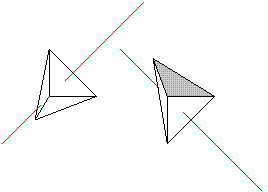Next: , Previous: Object transforms, Up: Introduction by example

### 2.6 Repeated objects

To draw seven instances of the tetrahedron, each differing from the last by the same transform, replace the last two commands of the previous example with

  repeat { 7, rotate(15, (0,0,0), [1,0,0]) % copy in new position
then translate([2,0,0]) } {pierced_tetrahedron}

And the result....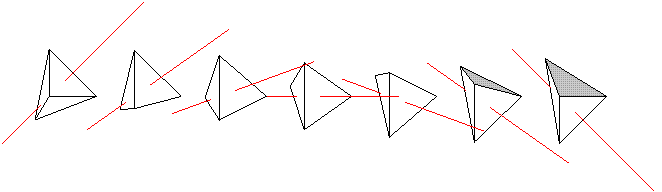Previous: Repeated objects, Up: Introduction by example

### 2.7 Swept objects

Many familiar shapes can be generated by sweeping simpler ones through space and considering the resulting path, surface, or volume. Sketch implements this idea in the sweep command.

  def n_segs 8
sweep { n_segs, rotate(180 / n_segs, (0,0,0), [0,0,1]) } (1,0,0)

This code sweeps the point (1,0,0) eight times by rotating it 180/8 = 22.5 degrees each time and connecting the resulting points with line segments. The def used here is a scalar definition. References to scalars have no enclosing brackets at all.

Next: , Previous: Swept objects, Up: Swept objects

#### 2.7.1 Point sweeps

Sweeping a point makes a one-dimensional path, which is a polyline. Since we have swept with a rotation, the result is a circular arc. Here is what it looks like.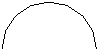This is the first example we have seen of sketch arithmetic. The expression 180 / n_segs causes the eight rotations to add to 180. If you're paying attention, you'll have already noted that there are nine points, producing eight line segments.

You can cause the swept point to generate a single polygon rather than a polyline by using the closure tag <> after the number of swept objects. Code and result follow

  def n_segs 8
sweep { n_segs<>, rotate(180 / n_segs, (0,0,0), [0,0,1]) } (1,0,0)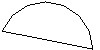Next: , Previous: Point sweeps, Up: Swept objects

#### 2.7.2 Polyline sweeps

Sweeping a polyline produces a surface composed of many faces. The unbroken helix in the example Helix with cull set false then true is produced by this code (plus a surrounding put rotation to make an interesting view; this has been omitted).

  def K [0,0,1]
sweep[cull=false] {
60,
rotate(10, (0,0,0), [K]) then translate(1/6 * [K])
} line[linewidth=2pt](-1,0)(1,0)

Again, 60 segments of the helix are produced by connecting 61 instances of the swept line. Options applied to the sweep, here cull=false, are treated as options for the generated polygon or polyline. Options of the swept line itself, here linewidth=2pt, are ignored, though with a warning. This def is a vector definition, which must be referenced with square brackets, e.g. [foo].

Next: , Previous: Polyline sweeps, Up: Swept objects

#### 2.7.3 Nested sweeps

When the center point of rotation is omitted, the origin is assumed. When a point has only two coordinates, they are taken as x and y, with z=0 assumed. A toroid is therefore obtained with this code.

  def n_toroid_segs 20   def n_circle_segs 16
def r_minor 1          def r_major 1.5
sweep { n_toroid_segs, rotate(360 / n_toroid_segs, [0,1,0]) }
sweep { n_circle_segs, rotate(360 / n_circle_segs, (r_major,0,0)) }
(r_major + r_minor, 0)

For intuition, the idea of the code is to sketch a circle to the right of the origin in the xy-plane, then rotate that circle “out of the plane” about the y-axis to make the final figure. This produces the following. (A view rotation and some axes have been added.)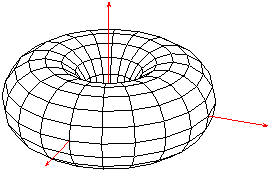This example also shows that the swept object may itself be another sweep. In fact, it may be any sketch expression that results in a list of one or more points or, alternately, a list of one or more polylines and polygons. The latter kind of list can be created with a { }-enclosed block, perhaps following a put or repeat.

Next: , Previous: Nested sweeps, Up: Swept objects

#### 2.7.4 Polygon sweeps

Sweeping a polygon creates a closed surface with polygons at the ends, which are just copies of the original, appropriately positioned. See Solid coil example. Options on the swept polygon, if they exist, are applied to the ends. Otherwise the sweep options are used throughout.

Next: , Previous: Polygon sweeps, Up: Swept objects

#### 2.7.5 Polyline sweeps with closure

A polyline sweep with a closure tag creates another kind of closed surface. First, the polyline segments are connected by faces, just as without the closure tag. Then, each set of end points is joined to make a polygon, one for each end. A code for several views of a cylindrical prism follows.

  def n_cyl_segs 20  def n_views 5  def I [1,0,0]
def endopts [fillcolor=lightgray]
repeat { n_views, rotate(180/n_views, [I]) then translate([I] * 2.1) }
sweep[endopts]{ n_cyl_segs<>, rotate(360/n_cyl_segs, [0,1,0]) }
line[fillcolor=white](1,-1)(1,1)

It produces this drawing.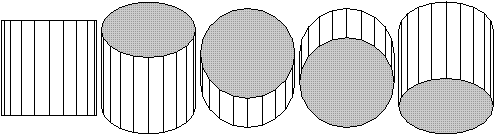The options of the swept line, if any, are applied to the faces produced by sweeping the line, but not the end polygons. Otherwise, the sweep options are applied throughout. The def in this example is an option definition. References to options must be enclosed in square brackets, e.g.  [foo]. Happily, the syntax of sketch is such that options references can never be confused with vector references. While not apparent in this example, options references are useful when defining many objects with a similar appearance.

Next: , Previous: Polyline sweeps with closure, Up: Swept objects

#### 2.7.6 Affine arithmetic

The arithmetic [I] * 2.1 above hints at a larger truth. Sketch operators work on scalars, vectors, points, and transforms according to the general rules of affine algebra. This can be helpful for setting up diagrams with computed geometry. For example, if you have triangle vertices (p1) through (p3) and need to draw a unit normal vector pointing out of the center of the triangle, this code does the trick.

  def p1 (1,0,0)  def p2 (0,0.5,0)  def p3 (-0.5,-1,2)
def O (0,0,0)
def N unit( ((p3) - (p2)) * ((p1) - (p2)) )
def n1 ((p1)-(O) + (p2)-(O) + (p3)-(O)) / 3 + (O)
def n2 (n1)+[N]
polygon(p1)(p2)(p3)
line[arrows=*->](n1)(n2)

The first line computes the cross product of two edge vectors of the triangle and scales it to unit length. The second computes the average of the vertices. Note that subtraction and addition of the origin effectively convert vectors to points and vice versa. The line command draws the normal at the correct spot.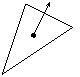Two caveats regarding this example remain. First, the only way to use PSTricks-style arrows is with arrows=. The alternative syntax for PSTricks arrows is not allowed in sketch. Second, you might like to eliminate the third def and write instead the following.

  line[arrows=*->](n1) (n1)+[N]

This is not allowed. The point lists in drawables may consist only of explicit points or point references. You may, however, use arithmetic to calculate point components. The following works, though it's a little cumbersome.
  line[arrows=*->](n1)((n1)'x+(N)'x, (n1)'y+(N)'y, (n1)'z+(N)'z)

Obviously, the tick operator 'x extracts components of points and vectors.

Previous: Affine arithmetic, Up: Swept objects

#### 2.7.7 More to learn

This is not the end of the story on sweeps! We invite the reader into the main body of this documentation Sweeps to learn more.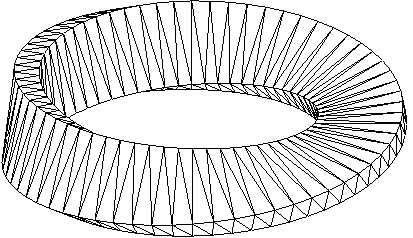Who knows where you'll finish?

Next: , Previous: Introduction by example, Up: Top

## 3 Input language

This chapter describes the sketch input language in detail.

Next: , Previous: Input language, Up: Input language

### 3.1 Basics

Sketch input is plain ASCII text, usually stored in an input file. It describes a scene, so the sketch language is a scene description language. Sketch input is also declarative. It merely declares what the scene ought to look like when drawing is complete and says very little about how sketch should do its work. Sketch commands are not executed sequentially as in the usual programming language. They merely contribute to that declaration.

A few syntactic details are important. Case is significant in the sketch language. With a few exceptions, white space is not. This includes line breaks. Comments begin with % or # and extend to the end of the line. You can disable a chunk of syntactically correct sketch code by enclosing it in a def. There is a simple “include file” mechanism. The command

  input{otherfile.sk}

causes the contents of otherfile.sk to be inserted as though they were part of the current file.

Next: , Previous: Language basics, Up: Language basics

#### 3.1.1 Identifiers

Identifiers in sketch are references to earlier-defined options, scalars, points, vectors, transforms, drawables, and tags. Definitions are explained in Definitions.

An identifier consists of a leading letter followed by letters, numbers and underscores. The last character may not be an underscore. Keywords cannot be used as identifiers, and reserved words ought to be avoided. See Key and reserved words.

Next: , Previous: Identifiers, Up: Language basics

#### 3.1.2 Key and reserved words

The keywords of sketch are picturebox curve def dots frame global input line polygon put repeat set sweep and then. The sketch parser will note a syntax error if any of these are used in place of a proper identifier.

In addition, there are reserved words that can currently be defined by the user, but with the risk that future versions of sketch will reject those definitions. The reserved words are atan2 cos inverse perspective project rotate scale sin special sqrt translate unit and view.

Next: , Previous: Key and reserved words, Up: Language basics

#### 3.1.3 Literals

Literals in sketch include scalars, points, vectors, and transforms. Literals, along with defined object references, are used in arithmetic expressions. See Arithmetic.

Next: , Previous: Literals, Up: Literals
##### 3.1.3.1 Scalar literals

Scalar literals are positive floating point numbers with syntax according to C conventions. The following are some examples.

     0 1004 .001 8.3143 3. 1.60E-19 6.02e+23


Scalar literals may not contain embedded spaces.

Next: , Previous: Scalar literals, Up: Literals
##### 3.1.3.2 Point and vector literals

Points and vector literals have these forms respectively.

     (X,Y,Z)  [X,Y,Z]


Each of the components is itself a scalar expression. The z-components are optional and default to zero.

Previous: Point and vector literals, Up: Literals
##### 3.1.3.3 Transform literals

Most transform literals are formed by constructors. These are summarized in the following table.

Constructor Param types Description
rotate(A,P,X) scalar,point,vector Rotate A degrees about point P with axis X according to the right hand rule. See Right hand rule. P and X are both optional and default to the origin and the z-axis respectively.
translate(X) vector Translate by X.
scale(S) scalar Scale uniformly by factor S.
scale(V) vector Scale along each axis by components of V.
project() Same as scale([1,1,0]).
project(S) scalar Perspective projection with view center at origin and projection plane z=-S.
perspective(S) scalar Perspective transform identical to project(S) except that the z-coordinate of the transformed result is pseudodepth, usable by the hidden surface algorithm.
view(E,D,U) point,vector,vector View transform similar to that of OpenGL's. The eye point E is translated to the origin while a rotation is also applied that makes the view direction vector D and the view “up” vector U point in the negative z- and the y-directions respectively. If U is omitted, it defaults to [0,1,0]. When U is omitted, D may be also; it defaults to (0,0,0)-(E), a vector pointing from the eye toward the origin.
view(E,L,U) point,point,vector An alternate form of view(E,D,U) above where the view direction parameter D is replaced with a “look at” point L, i.e., a point where the viewer is focusing her attention. This form of view is equivalent to view(E, (L)-(E), U), where (L)-(E) is a direction vector. U is optional and defaults to [0,1,0].
[[a_11,a_12,a_13,a_14]
 [a_21,a_22,a_23,a_24]
 [a_31,a_32,a_33,a_34]
 [a_41,a_42,a_43,a_44]]
16 scalars Direct transform matrix definition. Each of the a_ij is a scalar expression. If you don't know what this is about, you don't need it.
The project constructor is not generally useful because it defeats hidden surface removal by collapsing the scene onto a single plane. It is a special purpose transform for drawing pictures of scenes where three-dimensional objects are being projected onto planes. See, for example, Overview.

Next: , Previous: Literals, Up: Language basics

#### 3.1.4 Arithmetic expressions

Arithmetic expressions over sketch literals and defined identifiers are summarized in the following tables.

Next: , Previous: Arithmetic, Up: Arithmetic
##### 3.1.4.1 Two-operand (binary) forms and precedence

Most two-operand binary forms have meanings dependent on the types of their arguments. An exhaustive summary of the possibilities is given in the following table.

Left Op Right Result Description
scalar + scalar scalar Scalar sum.
vector + vector vector Vector sum.
point + vector point Point-vector affine sum.
vector + point " "
scalar - scalar scalar Scalar difference.
vector - vector vector Vector difference.
point - point vector Point-point affine difference.
point - vector point Point-vector affine difference.
scalar * or . scalar scalar Scalar product.
scalar * or . vector vector Scalar-vector product.
vector * or . scalar " "
vector * vector vector Vector cross-product.
vector . vector scalar Vector dot product.
scalar ^ scalar scalar Raise scalar to scalar power.
transform ^ integer transform Raise transform or integer power.
transform * or . point point Affine point transform (right-to-left).
transform * or . vector vector Affine vector transform (right-to-left).
transform * or . transform transform Transform composition (right-to-left).
point then transform point Affine point transform (left-to-right).
vector then transform vector Affine vector transform (left-to-right).
transform then transform transform Transform composition (left-to-right).
scalar / scalar scalar Scalar division.
vector / scalar vector Vector component-wise division by scalar.
point ' x, y, or z scalar Point component extraction.
vector ' x, y, or z scalar Vector component extraction.



Operator precedence is shown in this table.

Op Precedence
' highest (most tightly binding)
^
- (unary negation)
* . /
+ -
then lowest (least tightly binding)



All operations are left-associative except for ^. Parentheses ( ) are used for grouping to override precedence in the usual way.

As you can see, the dot operator . is usually a synonym for run-of-the-mill multiplication, *. The meanings differ only for vector operands. The then operator merely reverses the operand order with respect to normal multiplication *. The intent here is to make compositions read more naturally. The code

     (1,2,3) then scale(2) then rotate(30) then translate([1,3,0])


expresses a series of successive modifications to the point, whereas the equivalent form

     translate([1,3,0]) * rotate(30) * scale(2) * (1,2,3)


will be intuitive only to mathematicians (and perhaps Arabic language readers).

Previous: Two-operand (binary) forms, Up: Arithmetic
##### 3.1.4.2 Unary forms

Unary or one-operand forms are summarized in the following table, where X stands for the operand.

Op Operand Result Description
-X scalar scalar Unary scalar negation.
-X vector vector Unary vector negation.
|X| vector scalar Vector length.
unit(X) vector vector Unit vector with same direction.
sqrt(X) scalar scalar Scalar square root.
sin(X) scalar scalar Trigonometric sine (X in degrees).
cos(X) scalar scalar Trigonometric cosine (X in degrees).
atan2(X,Y) scalar scalar Polar angle in degrees of vector [X,Y].
inverse(X) transform transform Inverse transform.



Errors are reported when |X|, unit, sqrt, atan2, and inverse fail due to bad parameters.

Previous: Arithmetic, Up: Language basics

#### 3.1.5 Options

Syntax:

     [key1=val1,key2=val2,...]


Options are used to specify details of the appearance of drawables. As shown above, they are given as comma-separated key-value pairs.

Next: , Previous: Options, Up: Options
##### 3.1.5.1 PSTricks options

When language pstricks is selected (the default), permissible key-value pairs include all those for similar PSTricks objects. For example, a polygon might have the options

  [linewidth=1pt,linecolor=blue,fillcolor=cyan]

Sketch merely passes these on to PSTricks without checking or modification. Option lists are always optional. A missing options list is equivalent to an empty one [].

When a polygon has options for both its face and its edges, and the polygon is split by the hidden surface algorithm, sketch must copy the edge options to pslines for the edge segments and the face options to pspolygons. Options known to sketch for purposes of this splitting operation include arrows, dash, dotsep, fillcolor, fillstyle, linecolor, linestyle, linewidth, opacity, showpoints, strokeopacity, and transpalpha.

Next: , Previous: PSTricks options, Up: Options
##### 3.1.5.2 TikZ/PGF options

TikZ/PGF options are handled much as for PSTricks. Though TikZ/PGF often allows colors and styles to be given without corresponding keys, for example,

  \draw[red,ultra thick](0,0)--(1,1);

this is not permitted in sketch. To draw a red, ultra-thick line in sketch, the form is
  line[draw=red,style=ultra thick](0,0)(1,1)


Just as for PSTricks, when a polygon has options for both its face and its edges, and the polygon is split by the hidden surface algorithm, sketch must copy the edge options to pslines for the edge segments and the face options to pspolygons. TikZ/PGF options known to sketch for purposes of this splitting operation include arrows, cap, color, dash pattern, dash phase, double distance, draw, draw opacity, fill, fill opacity, join, line width, miter limit, pattern, pattern color, and style.

The style option can contain both face and edge information, so sketch must check the style value. Values known to sketch include dashed, densely dashed, densely dotted, dotted, double, loosely dashed, loosely dotted, nearly opaque, nearly transparent, semithick, semitransparent, solid, thick, thin, transparent, ultra nearly transparent, ultra thick, ultra thin, very nearly transparent, very thick, and very thin.

Next: , Previous: TikZ/PGF options, Up: Options
##### 3.1.5.3 Dots in TikZ/PGF

TikZ/PGF does not have a dots command as does PSTricks. Instead, Sketch emits dots as filldraw circles. The diameter may be set using the option dotsize borrowed from PSTricks. The dotsize option will be removed from the option list in the output filldraw command. Other options work in the expected way. For example, fill sets fill color and color sets line color of the circles.

Next: , Previous: Dots in TikZ/PGF, Up: Options
##### 3.1.5.4 TikZ/PGF user-defined styles

TikZ/PGF allows named styles defined by the user, for example

  \tikzstyle{mypolygonstyle} = [fill=blue!20,fill opacity=0.8]
\tikzstyle{mylinestyle} = [red!20,dashed]

Since sketch has no information on the contents of such styles, it omits them entirely from lines, polygons, and their edges during option splitting. For example,
  polygon[style=mypolygonstyle,style=thick](0,0,1)(1,0,0)(0,1,0)
line[style=mylinestyle](-1,-1,-1)(2,2,2)

produces the TikZ output
  \draw(-1,-1)--(.333,.333);
\filldraw[thick,fill=white](0,0)--(1,0)--(0,1)--cycle;
\draw(.333,.333)--(2,2);

Note that the user-defined styles are not present. Sketch also issues warnings:
  warning, unknown polygon option style=mypolygonstyle will be ignored
warning, unknown line option style=mylinestyle will be ignored


The remedy is to state explicitly whether a user-defined style should be attched to polygons or lines in the TikZ output using pseudo-options fill style and line style,

  polygon[fill style=mypolygonstyle,style=thick](0,0,1)(1,0,0)(0,1,0)
line[line style=mylinestyle](-1,-1,-1)(2,2,2)

Now, the output is
  \draw[mylinestyle](-1,-1)--(.333,.333);
\filldraw[mypolygonstyle,thick](0,0)--(1,0)--(0,1)--cycle;
\draw[mylinestyle](.333,.333)--(2,2);


A useful technique is to include user-defined style definitions in sketch code as specials with option [lay=under] to ensure that the styles are emitted first in the output, before any uses of the style names. 2 For example,

  special|\tikzstyle{mypolygonstyle} = [fill=blue!20,fill opacity=0.8]|[lay=under]
special|\tikzstyle{mylinestyle} = [red!20,dashed]|[lay=under]

The author is responsible for using the key, line style or fill style, that matches the content of the style definition.

Next: , Previous: TikZ/PGF user-defined styles, Up: Options
##### 3.1.5.5 Transparency

Both PSTricks and TikZ/PGF support polygon options that have the effect of making the polygon appear transparent. For PSTricks, keywords opacity and transpalpha have both been used, with the correct one depending on version. TikZ/PGF uses opacity only. When transparent polygons are in the foreground, objects behind them (drawn earlier) are visible with color subdued and tinted. The hidden surface algorithm of sketch works well with such transparent polygons.

Note that cull=false must be used for rear-facing polygons to be visible when positioned behind other transparent surfaces.

Previous: Transparency, Up: Options
##### 3.1.5.6 Internal options

There are also internal options used only by sketch and not passed on to PSTricks. These are summarized in the following table.

Key Possible values Description
cull true, false Turn culling of backfaces on and off respectively for this object. The default value is true.
lay over, in, under Force this object to be under or over all other objects in the depth sort order created by the hidden surface algorithm. The default value over guarantees that output due to the special will be visible.
split true, false Turn splitting of sweep-generated body polygons on and off respectively. See Sweeps. The default value true causes “warped” polygons to be split into triangles, which avoids mistakes by the hidden surface algorithm.

#### 3.1.6 Point lists

Syntax:

     (x1,y1,z1)(x2,y2,z2)...


A sequence of one or more points makes a point list, a feature common to all drawables. Each of the point components is a scalar arithmetic expression. Any point may have the z-component omitted; it will default to z=0.

Next: , Previous: Language basics, Up: Input language

### 3.2 Drawables

Drawables are simply sketch objects that might appear in the drawing. They include dots, polylines, curves, polygons, and more complex objects that are built up from simpler ones in various ways. Finally, special objects are those composed of LaTeX or PSTricks code, perhaps including coordinates and angles computed by sketch.

Next: , Previous: Drawables, Up: Drawables

#### 3.2.1 Dots

Syntax:

     dots[options] point_list


This command is the three-dimensional equivalent of the PSTricks command \psdots.

Next: , Previous: Dots, Up: Drawables

#### 3.2.2 Lines

Syntax:

     line[options] point_list


This command is the three-dimensional equivalent of the PSTricks command \psline.

Next: , Previous: Lines, Up: Drawables

#### 3.2.3 Curves

Syntax:

     curve[options] point_list


This command is the three-dimensional equivalent of the PSTricks command \pscurve. It is not implemented in the current version of sketch.

Next: , Previous: Curves, Up: Drawables

#### 3.2.4 Polygons

Syntax:

     polygon[options] point_list


This command is the three-dimensional equivalent of the PSTricks command \pspolygon. The sketch hidden surface algorithm assumes that polygons are convex and planar. In practice, drawings may well turn out correctly even if these assumptions are violated.

Next: , Previous: Polygons, Up: Drawables

#### 3.2.5 Specials

Syntax:

     special $raw_text$[lay=lay_value] point_list


Here $ can be any character and is used to delimit the start and end of raw_text. The command embeds raw_text in the sketch output after performing substitutions as follows. • #i where i is a positive integer is replaced by the i'th point in point_list. • #{i} is also replaced as above. • #i-j where i and j are positive integers is replaced by a string {angle} where angle is the polar angle of a vector from the i'th point in point_list to the j'th. • #{i-j} is also replaced as above. • ## is replaced with #. The forms with braces { } are useful when the argument is immediately followed by a digit that is part of the TeX code. The only useful option of special is lay. See Internal options. Next: , Previous: Specials, Up: Drawables #### 3.2.6 Sweeps Syntax:  sweep { n, T_1, T_2, ..., T_r }[options] swept_object sweep { n<>, T_1, T_2, ..., T_r }[options] swept_object  The sweep connects n (or perhaps n+1) copies of swept_object in order to create a new object of higher dimension. The T_i (for i between 1 and r) are transforms. The k'th copy of swept_object is produced by applying the following transform to the original.  T_1^k then T_2^k then ... then T_r^k  Here T^k means “transform T applied k times.” The original object is the zero'th copy, with k=0 and effectively no transform applied (T^0=I, the identity transform). The method of connecting the copies depends on the type of swept_object and on whether the closure tag <> is present or not. An example of a sweep where r=2 is the Mobius figure at More to learn. Next: , Previous: Sweeps, Up: Sweeps ##### 3.2.6.1 Swept points If swept_object is a point list and there is no closure tag, then sweep connects n+1 successive copies of each point (including the original) with straight line segments to form a polyline. If there are m points in the original point list, then m polylines with n segments each are formed by the sweep. In this manner, sweep forms a set of one-dimensional objects (polylines) from zero-dimensional ones (points). When there is a closure tag, sweep connects n successive copies of each point (including the original) with straight line segments and finally connects the last copy back to the original to form a polygon with n sides. If there are m points in the original point list, then m polygons with n sides each are formed by the sweep. In this manner, sweep forms a set of two-dimensional objects (polygons) from zero-dimensional ones (points). Options of the sweep are copied directly to the resulting polyline(s). Next: , Previous: Swept points, Up: Sweeps ##### 3.2.6.2 Swept lines If swept_object is a polyline and there is no closure tag, then sweep connects n+1 successive copies of the polyline (including the original) with four-sided polygons, each pair of copies giving rise to a “polygon strip.” If there are m points in the original polyline, then (m-1)n polygons are formed by the sweep. We call these body polygons. In this manner, sweep forms a two-dimensional surface from from a one-dimensional polyline. The order of vertices produced by sweep is important. If a polygon's vertices do not appear in counter-clockwise order in the final image, the polygon will be culled (unless cull=false is set). If the points in the k'th copy of the polyline are P_1, P_2, ..., P_m, and the points in the next copy, the (k+1)st, are P_1', P_2', ..., P_m', then the vertex order of the generated polygons is  Body polygon 1: P_2 P_1 P_1' P_2' Body polygon 2: P_3 P_2 P_2' P_3' ... Body polygon m-1: P_m P_m-1 P_m-1' P_m'  Options of unclosed line sweeps are copied to each output polygon. Options of the swept line are ignored. When there is a closure tag, then sweep connects n successive copies of the polyline (including the original) with four-sided body polygons just as the case with no closure tag. It then connects the last copy back to the original to form a ribbon-shaped surface that closes on itself with two holes remaining. Finally, the sweep adds two more polygons to seal the holes and form a closed surface that, depending on the sweep transforms, may represent the boundary of a solid. In this manner, sweep forms the boundary of a three-dimensional object from a one-dimensional polyline. We call these hole-filling polygons ends. The order of vertices of end polygons is important for correct culling as described above. If P_1^1, P_1^2, ..., P_1^n are the n copies of the first polyline point and P_m^1, P_m^2, ... ,P_m^n are the n copies of the last polyline point, then the end polygon vertex order is  End polygon 1: P_1^n, P_1^n-1, ... ,P_1^1 End polygon 2: P_m^1, P_m^2, ... ,P_m^n  If there are no options on the swept line, then the sweep options are copied to each output polygon. If the swept line does have options, these are copied to corresponding body polygons; the sweep options are copied to the end polygons. In this manner, body and ends may be drawn with different characteristics such as fillcolor. Next: , Previous: Swept lines, Up: Sweeps ##### 3.2.6.3 Swept polygons If swept_object is a polygon, the sweep connects n+1 successive copies of the closed polyline border of the polygon to form body polygons exactly as though the border were a swept polyline as described in Swept lines. If there are m points in the original polygon, then mn body polygons are formed by this sweep. The body polygons form an extrusion of the boundary of the original polygon with two holes at the open ends. Finally, the sweep adds two copies of the original polygon to cover the holes. We call these hole-filling polygons ends. In this manner, sweep forms the boundary of a three-dimensional object from a two-dimensional polygon. The order of vertices of end polygons is important for correct culling as described above. An exact copy of the original polygon with vertex order intact forms the first end polygon. The other end polygon results from transforming and the reversing the order of vertices in the original. The transform places the original polygon at the uncovered hole; it is  T_1^n then T_2^n then ... then T_r^n.  If there are no options on the swept polygon, then the sweep options are copied to each output polygon. If the swept polygon does have options, these are copied to the ends; the sweep options are copied to the body polygons. In this manner, body and ends may be drawn with different characteristics such as fillcolor. Next: , Previous: Swept polygons, Up: Sweeps ##### 3.2.6.4 Swept blocks The swept object swept_object may also be any collection of polylines and polygons. This may be a block composed of line and/or polygon commands in braces { }, or it may be the result of a repeat, another sweep, etc. The sweep acts independently on each object in the block exactly as if it were a single swept object described above in Swept lines and Swept polygons. Previous: Swept blocks, Up: Sweeps ##### 3.2.6.5 Sweep face splitting Before sending each four-sided body polygon of a sweep to the output, sketch tests to see if it is roughly planar. Since planarity is necessary for proper functioning of the hidden surface algorithm, “warped” polygons are automatically split into two triangles. Hole-filling polygons produced by closure-tagged line sweeps are not split. Nor are original polygons in polygon sweeps. It is the user's responsibility to ensure these are planar. Next: , Previous: Sweeps, Up: Drawables #### 3.2.7 Blocks Any sequence of drawables may be grouped in a block merely by enclosing them in braces { }. A block is itself drawable. A key use of blocks is to extend the effect of a single def, Definitions, put Puts, sweep Sweeps, or repeat Repeats to include several objects rather than one. Definitions (See Definitions.) inside a block have lexical scope extending from the place of definition to the end of the block. Next: , Previous: Blocks, Up: Drawables #### 3.2.8 Repeats Syntax:  repeat { n, T_1, T_2, ..., T_r } repeated_object  The repeat makes n transformed copies of repeated_object (including the original). The T_i are transforms. The k'th copy of the repeated_object (for k=0,1,...,n-1) is produced in the same manner as for sweeps described in Sweeps. This is repeated here (no pun intended) for convenience. To make the k'th copy, the following transform is applied to the original object.  T_1^k then T_2^k then ... then T_r^k  Here T^k means “transform T applied k times.” Previous: Repeats, Up: Drawables #### 3.2.9 Puts Syntax:  put { T } put_object  Put merely applies transform T to the drawable put_object. Next: , Previous: Drawables, Up: Input language ### 3.3 Definitions Definitions give names to sketch objects. Definitions alone are benign. A sketch input file consisting entirely of definitions will generate no drawing. Only when definitions are referenced do they potentially lead to ink on the drawing. The intent of definitions is to make sketch code more concise and readable. There is no input file employing definitions that could not be re-written without them. Definable objects include any result of an affine arithmetic expression (scalar, point, vector, or transform), any drawable object (dots, line, curve, polygon, block, sweep, put, repeat, or special), and option strings. In addition, tag definitions, which have no associated object at all, allow the meaning of other definitions to be selected from a set of alternatives. Since tags may be defined (and undefined) in the command line of sketch, they can be an aid in the script-driven preparation of documents. Next: , Previous: Definitions, Up: Definitions #### 3.3.1 Forms of definitions Definitions have three possible forms, simple, with alternatives, and tag as shown here in order. Syntax:  def id object % simple def def id <tag_1> object_1 % def with alternatives <tag_2> object_2 ... <> default_object def id <> % tag def  The simple definition merely associates object with the identifier id. The definition with alternatives associates object_i with id, where tag_i is the first defined tag in the list of alternative tag references. If no tag in the list is defined, then default_object is associated with identifier id. The final form defines id as a tag. Another way to define a tag is with the -D command line option. See Command line. Previous: Forms of definitions, Up: Definitions #### 3.3.2 Forms of references References to defined names are enclosed in bracketing delimiters. The delimiter characters imply the type of the associated value as shown in the table below. A type error is raised if the type of a reference does not match the type of the defined value. The intent of this mechanism is, again, to make sketch input files more readable. Type Reference scalar id point (id) vector [id] transform [[id]] drawable {id} options [id] or [id1,...,idN] tag <id>   Note that square brackets [ ] are used both for vector and for options references. Details of sketch syntax make it impossible for these two reference types to be confused. The special multiple reference [id1,id2,...,idN] acts as if the respective lists of options were concatenated. Previous: Definitions, Up: Input language ### 3.4 Global environment An optional global environment block provides a few ways to affect the entire scene. The block must appear as the last text in the sketch input file. It may include definitions, but note that previous definitions at the top level (not nested inside blocks) are also available. Syntax:  global { environment_settings }  The contents of environment_settings are discussed in the sections that follow. Next: , Previous: Global environment, Up: Global environment #### 3.4.1 Global options Syntax:  set [ options ]  The contents of options, except for sketch internal options, are copied as-is to a \psset that appears before anything else in the output file. This is a good place to set unit, a default linewidth, etc. Internal options work on all objects where they make sense. This includes cull and split (but not lay). See Internal options. Next: , Previous: Global options, Up: Global environment #### 3.4.2 Camera Syntax:  camera transform_expression  The transform_expression is applied after all other transformations of the scene. This is currently only useful for transforming the bounding box. See Picture box. It will play a role in any future implementation of clipping. Next: , Previous: Camera, Up: Global environment #### 3.4.3 Picture box Syntax:  picturebox[baseline] picturebox[baseline] (p1)(p2)  The first form of picturebox causes a scalar baseline fraction to be emitted in the pspicture environment of the output. See PSTricks documentation for pspicture. In the second form, the baseline fraction is optional, and the two points that follow define the diagonal of a three-dimensional bounding box for the completed scene. The parallel projection of the bounding box determines the corners of the drawing's pspicture* environment, which is used in place of pspicture. This causes PostScript to clip the final drawing to the bounding box in 2d. If there is a camera specified, the camera tranformation is applied to the bounding box, and the pspicture is set just large enough to include the transformed box. When no bounding box is given, sketch computes one automatically. Next: , Previous: Picture box, Up: Global environment #### 3.4.4 Frame Syntax:  frame [options]  Causes a \psframebox to surround the pspicture environment in the output. If options are present, they are copied as-is. Normally one would want to set linewidth, linestyle, linecolor, etc. If omitted, then framesep=0pt is added so that the frame tightly hugs the pspicture. Previous: Frame, Up: Global environment #### 3.4.5 Language  language tikz language tikz, context language pstricks language pstricks, latex  Sets the output language generated by sketch. The set of options understood by sketch also changes. For example, the PSTricks option linewidth will not be properly handled if language is set to tikz. Similarly, the TikZ option line style (note the space) will not be properly handled if language is set to pstricks. If no language is specified, the default pstricks is used. An optional comma followed by latex or context specifies the macro package that the output should assume. This affects the picture environment commands emitted and the document template used with the -T option. See Command line. Note that at the time this manual was generated, PSTricks was not supported by LaTeX or by ConTeXt. Next: , Previous: Input language, Up: Top ## 4 Building a drawing Successful drawings with sketch and with any scene description language require that the user develop an accurate mental picture of her code and its meaning. This image is best built in small pieces. Therefore, sketch inputs are best created in small increments with frequent pauses to compile and view the results. Careful comments in the input often help as a scene grows in complexity. Next: , Previous: Building a drawing, Up: Building a drawing ### 4.1 Overview As an overview, let's develop a diagram that shows how a perspective projection transform works. We'll start with the traditional reference object used in computer graphics textbooks, a house-shaped prism. Begin by defining the points of the house. Rather than defining the faces of the house as polygons and transforming those, we are going to transform the points themselves with sketch arithmetic so that we have names for the transformed points later.  % right side (outside to right) def R1 (1,1,1) def R2 (1,-1,1) def R3 (1,-1,-1) def R4 (1,1,-1) def R5 (1,1.5,0) % left side (outside to right--backward) def W [2,0,0] def L1 (R1)-[W] def L2 (R2)-[W] def L3 (R3)-[W] def L4 (R4)-[W] def L5 (R5)-[W]  To add a door to the house, we use a polygon slightly in front of the foremost face of the house.  % door def e .01 def D1 (0,-1,1+e) def D2 (.5,-1,1+e) def D3 (.5,0,1+e) def D4 (0,0,1+e)  Now let's create a new set of points that are a to-be-determined transform of the originals.  def hp scale(1) % house positioner def pR1 [[hp]]*(R1) def pR2 [[hp]]*(R2) def pR3 [[hp]]*(R3) def pR4 [[hp]]*(R4) def pR5 [[hp]]*(R5) def pL1 [[hp]]*(L1) def pL2 [[hp]]*(L2) def pL3 [[hp]]*(L3) def pL4 [[hp]]*(L4) def pL5 [[hp]]*(L5) def pD1 [[hp]]*(D1) def pD2 [[hp]]*(D2) def pD3 [[hp]]*(D3) def pD4 [[hp]]*(D4)  Note the use of a transform definition and transform references. Now define the seven polygonal faces of the house and the door using the transformed points as vertices. Be careful with vertex order!  def rgt polygon (pR1)(pR2)(pR3)(pR4)(pR5) def lft polygon (pL5)(pL4)(pL3)(pL2)(pL1) def frt polygon (pR2)(pR1)(pL1)(pL2) def bck polygon (pR4)(pR3)(pL3)(pL4) def tfr polygon (pR1)(pR5)(pL5)(pL1) def tbk polygon (pR5)(pR4)(pL4)(pL5) def bot polygon (pR2)(pL2)(pL3)(pR3) def door polygon[fillcolor=brown] (pD1)(pD2)(pD3)(pD4) def house { {rgt}{lft}{frt}{bck}{tfr}{tbk}{bot}{door} }  Time for a sanity check. Add the line  {house}  and this is what we get.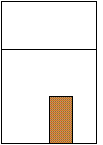This is correct, but does not reveal very much. Common errors are misplaced vertices and polygons missing entirely due to incorrect vertex order. To rule these out, let's inspect all sides of the house. This is not hard. Merely replace the reference {house} with a repeat. See Repeats.  repeat { 13, rotate(30, [1,2,3]), translate([3,0,0]) } {house}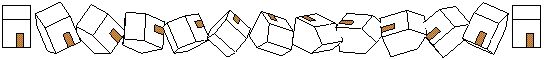Again things look correct. Note that the hidden surface algorithm handles intersecting polygons correctly where some copies of the house overlap. Let's lay out the geometry of perspective projection of the house onto a plane with rays passing through the origin. Begin by positioning the house twelve units back on the negative z-axis and adding a set of coordinate axes. To move the house we need only change the “house positioning” transform defined earlier.  def hp rotate(-40, [0,1,0]) then translate([0,0,-12]) def axes { def sz 1 line [arrows=<->] (sz,0,0)(O)(0,sz,0) line [arrows=->] (O)(0,0,sz) line [linewidth=.2pt,linecolor=blue,linestyle=dashed] (O)(0,0,-10) special |\uput[r]#1{$x$}\uput[u]#2{$y$}\uput[l]#3{$z$}| (sz,0,0)(0,sz,0)(0,0,sz) }  Time for another test. Let's build a real view transform, creating a virtual camera to look at the scene we are constructing. Replace the repeat with  def eye (10,4,10) def look_at (0,0,-5) put { view((eye), (look_at)) } { {house}{axes} }  The view transform repositions the scene so that the point eye is at the origin and the direction from eye to look_at is the negative z-axis. This requires a rotation and a translation that are all packed into the constructor view.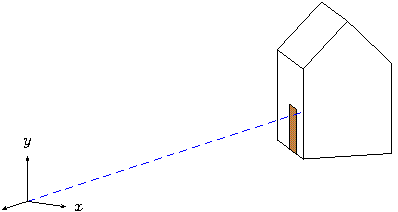This is starting to look good! Add the projection plane half way between the origin and the house at z=-5. We'll try the angle argument feature of special to position a label.  def p 5 % projection distance (plane at z=-p) def projection_plane { def sz 1.5 polygon (-sz,-sz,-p)(sz,-sz,-p)(sz,sz,-p)(-sz,sz,-p) special |\rput[b]#1-2#3{\footnotesize\sf projection plane}| (-sz,-sz,-p)(sz,-sz,-p)(0,-sz+.1,-p) }  Add {projection_plane} to the list of objects in the put above.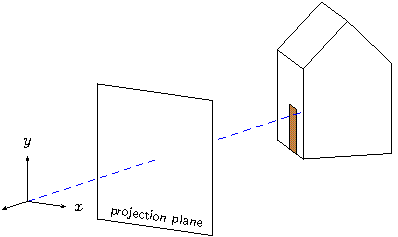The way we constructed the points of the house now makes it easy to draw rays of projection. We'll cast one ray from every visible vertex of the house and define options so the appearance of all rays can be changed at the same time.  def projection_rays { def rayopt [linewidth=.3pt,linecolor=lightgray] line [rayopt](O)(pR1) line [rayopt](O)(pR2) line[rayopt](O)(pR3) line [rayopt](O)(pR4) line [rayopt](O)(pR5) line [rayopt](O)(pL1) line [rayopt](O)(pL2) line[rayopt](O)(pL5) line [rayopt](O)(pD1) line [rayopt](O)(pD2) line [rayopt](O)(pD3) line [rayopt](O)(pD4) }  The result is shown here.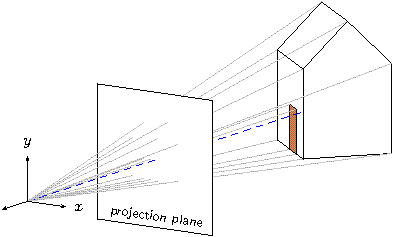The rays pierce the projection plane at the corresponding points on the perspective image we are trying to draw. Albrecht Dürer and his Renaissance contemporaries had the same idea in the early 1500's.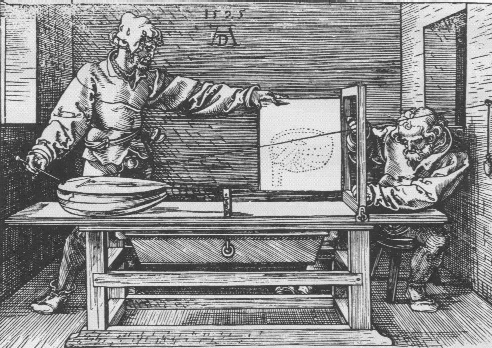All that's left is to find a way to connect the points of the house on the projection plane. We could pull out a good computer graphics text, find the necessary matrix, and enter it ourselves as a transform literal. See Transform literals. That work is already done, however. We can use the project(p) constructor. There are still some details that require care. Projection will flatten whatever is transformed onto the plane z=-p. Therefore any part of the house could disappear behind the projection plane (the hidden surface algorithm orders objects at the same depth arbitrarily). The door may also disappear behind the front of the house. To make sure everything remains visible, we'll place the house a tiny bit in front of the projection plane and a second copy of the door in front of the house.  def projection { % e is a small number defined above put { project(p) then translate([0,0,1*e]) } {house} put { project(p) then translate([0,0,2*e]) } {door} }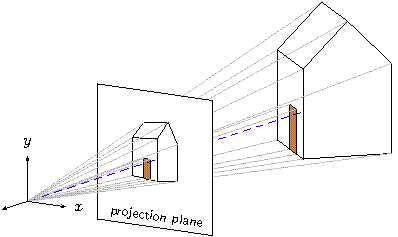If you have studied and understand all this, you are well on the way to success with sketch. Not shown are the 20 or so iterations that were required to find a reasonable viewing angle and house position, etc. Nonetheless, this drawing was completed in about an hour. While a GUI tool may have been a little faster, it is unlikely that a new drawing, itself a perspective projection of the scene, could be generated with two more minutes' work! Just change the view transform to  put { view((eye), (look_at)) then perspective(9) } { ...  and produce this.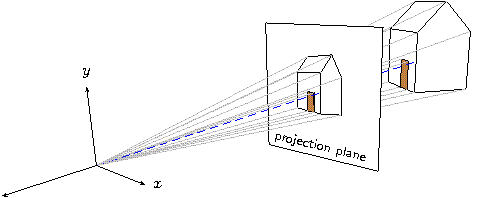Next: , Previous: Overview, Up: Building a drawing ### 4.2 A technical drawing Let's look at a drawing that represents the kind of problem sketch was meant to solve—a pair of textbook figures regarding a polygonal approximation of a truncated cone. Here are the pictures we will produce.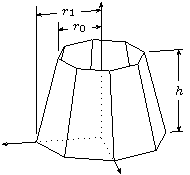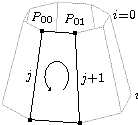The cone shape is just a swept line with no closure tag and culling turned off. Begin by setting up some useful constants.  def O (0,0,0) def I [1,0,0] def J [0,1,0] def K [0,0,1] def p0 (1,2) def p1 (1.5,0) def N 8 def seg_rot rotate(360 / N, [J])  The points p0 and p1 are the end points of the line to be swept. The definition seg_rot is the sweep transformation. With these, the cone itself is simple.  sweep[cull=false] { N, [[seg_rot]] } line(p0)(p1)  The axes are next and include an interesing trick that shows the hidden parts as dotted lines. The secret is draw the axes twice—solid lines with the normal hidden surface algorithm in effect, and then dotted with the option lay=over so that no polygons can hide them.  def ax (dx,0,0) % tips of the axes def ay (0,dy,0) def az (0,0,dz) line[arrows=<->,linewidth=.4pt](ax)(O)(ay) line[arrows=->,linewidth=.4pt](O)(az) % repeat dotted as an overlay to hint at the hidden lines line[lay=over,linestyle=dotted,linewidth=.4pt](ax)(O)(ay) line[lay=over,linestyle=dotted,linewidth=.4pt](O)(az) special|\footnotesize \uput[d]#1{$x$}\uput[u]#2{$y$}\uput[l]#3{$z$}| (ax)(ay)(az)  The labels are applied with PSTricks special objects as usual. For the height dimension mark, the power of affine arithetic is very helpful.  def hdim_ref unit((p1) - (O)) then [[seg_rot]]^2 def c0 (p0) then scale([J]) def h00 (c0) + 1.1 * [hdim_ref] def h01 (c0) + 1.9 * [hdim_ref] def h02 (c0) + 1.8 * [hdim_ref] line(h00)(h01) def h10 (O) + 1.6 * [hdim_ref] def h11 (O) + 1.9 * [hdim_ref] def h12 (O) + 1.8 * [hdim_ref] line(h10)(h11) line[arrows=<->](h02)(h12) def hm2 ((h02) - (O) + (h12) - (O)) / 2 + (O) special|\footnotesize\rput*#1{$h$}|(hm2)  The general idea employed here is to compute a unit “reference vector” parallel to the xz-plane in the desired direction of the dimension from the origin. The transformation [[seg_rot]]^2 rotates two segments about the y-axis. When applied to (p1) - (O), the resulting vector points to the right as shown. In this manner, we can pick any vertex as the location of the height dimension lines by varying the exponent of [[seg_rot]]. This is only one of many possible strategies. The computation of hm2 is a useful idiom for finding the centroid of a set of points. The two radius marks are done similarly, so we present the code without comment.  % radius measurement marks def gap [0,.2,0] % used to create small vertical gaps % first r1 def up1 [0,3.1,0] % tick rises above dimension a little def r1 ((p1) then [[seg_rot]]^-2) + [up1] def r1c (r1) then scale([J]) def r1t (r1) + [gap] def r1b ((r1t) then scale([1,0,1])) + [gap] line[arrows=<->](r1c)(r1) % dimension line line(r1b)(r1t) % tick def r1m ((r1) - (O) + (r1c) - (O)) / 2 + (O) % label position special |\footnotesize\rput*#1{$r_1$}|(r1m) % label % same drill for r0, but must project down first def up0 [0,2.7,0] def r0 ((p0) then scale([1,0,1]) then [[seg_rot]]^-2) + [up0] def r0c (r0) then scale([J]) def r0t (r0) + [gap] def r0b ((p0) then [[seg_rot]]^-2) + [gap] line[arrows=<->](r0c)(r0) line(r0b)(r0t) def r0m ((r0) - (O) + (r0c) - (O)) / 2 + (O) special |\footnotesize\rput*#1{$r_0\$}|(r0m)


The second drawing uses the same techniques. Only the method for drawing the elliptical arc is new. Here is the code.

  def mid ((p00)-(O)+(p10)-(O)+(p11)-(O)+(p01)-(O))/4+(O)
special|\rput#1{\pscustom{
\scale{1 1.3}
\psarc[arrowlength=.5]{->}{.25}{-60}{240}}}|
[lay=over](mid)

We could have swept a point to make the arc with sketch, but using a PSTricks custom graphic was simpler. Again we computed the centroid of the quadrilateral by averaging points. Note that scaling in Postscript distorts the arrowhead, but in this case the distortion actually looks better in the projection of the slanted face. A sketch arrowhead would not have been distorted.

The complete code for this example, which draws either figure depending on the definition of the tag <labeled>, is included in the sketch distribution in the file truncatedcone.sk.

Next: , Previous: A technical drawing, Up: Building a drawing

### 4.3 A hierarchical model

While sketch was never meant to be a geometric modeling language, it comes fairly close. The following example puts all we have seen to work in a very simple model of the human hand. Start by sweeping a line to make a truncated cone, which will be copied over and over again to make the segments of fingers.

  def O (0,0,0) % origin
def I [1,0,0] def J [0,1,0] def K [0,0,1] % canonical unit vectors
def segment {
def n_faces 8
sweep { n_faces<>, rotate(360 / n_faces, [J]) }
line(proximal_rad, 0)(distal_rad, distal_len)
}

In hand anatomy, distal is “at the tip” and proximal is “in the area of the palm.” We have omitted all the scalar constants. You can find them in hand.sk, which is provided in the sketch distribution.

We also need a prototypical sphere to use for the joints themselves.

  def joint_sphere {
def n_joint_faces 8
sweep [fillcolor=red] { n_joint_faces, rotate(360 / n_joint_faces, [J]) }
sweep { n_joint_faces, rotate(180 / n_joint_faces) }
(0, -joint_rad)
}


We'll now design the index finger (number 1 in our notational convention; finger 0 is the thumb). The distal rotation for the finger applies only to the tip, so we define the following.

  def distal_1 {
put { translate(joint_gap * joint_rad * [J])
then rotate(distal_1_rot, [I])
then translate((distal_len + joint_gap * joint_rad) * [J]) }
{segment}
put { rotate(distal_1_rot / 2, [I])
then translate((distal_len + joint_gap * joint_rad) * [J]) }
{joint_sphere}
put { scale( [J] + proximal_distal_ratio * ([I]+[K]) ) }
{segment}
}

The identifiers here are for size and location constants. The exception is distal_rot_1. This rotation parameter models the flexing of the finger tip. The first put makes a copy of the finger segment that is translated upward just far enough to make room for the spherical joint. Then it applies the distal rotation. Finally it translates the whole assembly upward again to make room for the middle phlanges (the next bone toward the palm). The second put positions the sphere. There is a rotation to place the grid on the sphere surface at an nice angle, then a translation to the base of the distal phlanges, which is also center of its rotation. Finally, the last put positions the middle segment itself.

The middle joint is the next one down, with rotation angle middle_rot_1. When this angle changes, we need all the objects in distal_1 to rotate as a unit. This is the reasoning behind the next definition.

  def finger_1 {
put { translate(joint_gap * joint_rad * [J])
then rotate(middle_1_rot, [I])
then translate((middle_ratio * distal_len +
joint_gap * joint_rad) * [J]) }
{distal_1}
put { scale(proximal_distal_ratio)
then rotate(middle_1_rot / 2, [I])
then translate((middle_ratio * distal_len +
joint_gap * joint_rad) * [J]) }
{joint_sphere}
put { scale( middle_ratio * [J] +
proximal_distal_ratio^2 * ([I]+[K]) ) }
{segment}
}

This looks very similar to the previous definition, and it is. The important difference is that rather than positioning and rotating a single segment, we position and rotate the entire “assembly” defined as distal_1. The rest is just arithmetic to compute sizes and positions that look nice. The last put places an appropriately shaped segment that is the proximal phlanges, the bone that joins the palm of the hand. This completes the finger itself.

All the other fingers are described identically to this one. We account for the fact that real fingers are different sizes in the next step, which is to build the entire hand.

The hand definition that follows includes a section for each finger. We'll continue with finger 1 and omit all the others. (Of note is that the thumb needs slightly special treatment—an extra rotation to account for its opposing angle. This is clear in the full source code.) Not surprisingly, the hand definition looks very much like the previous two. It should be no surprise that when the rotation parameter meta_1_rot changes, the entire finger rotates! There is an additional rotation that allows the fingers to spread laterally. We say these joints of the proximal phlanges have two degrees of freedom. The joints higher on the finger have only one. Finally, each finger is scaled by a factor to lend it proportion.

  def hand {
% finger 1 [all other fingers omitted]
def scale_1 .85
put { scale(scale_1)
then translate((joint_gap * joint_rad) * [J])
then rotate(meta_1_rot, [I])
then rotate(-spread_rot, [K])
then translate((proximal_1_loc) - (O)) }
{finger_1}
put { scale(scale_1 * proximal_distal_ratio^2)
then rotate(meta_1_rot / 2, [I])
then rotate(-spread_rot, [K])
then translate((proximal_1_loc) - (O)) }
{joint_sphere}

% palm
sweep { 1, rotate(6, (0,15,0), [I]) }
put { rotate(-3, (0,15,0), [I]) } {
polygon(proximal_1_loc)(proximal_2_loc)
(proximal_3_loc)(proximal_4_loc)
(h5)(h6)(h6a)(h9)(h10)
polygon(h6a)(h7)(h8)(h9)
}  }

The last section of the definition creates the polytope for the palm of the hand by sweeping a 10-sided polygon through a very short arc (9 degrees). This provides a wedge-shaped profile when viewed from the side. The thick end of the wedge is the wrist. Because the polygon is concave, it is split into into two convex shapes with nine and four vertices.

We can now have fun positioning the hand by adjusting the various rotation angles. The complete source includes definitions with alternatives that include the following views and more.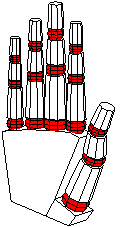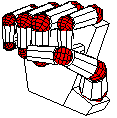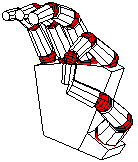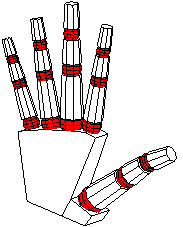Previous: A hierarchical model, Up: Building a drawing

### 4.4 Caveats

Sketch is a fairly powerful tool for drawing, but, just as with TeX, the power to create beautiful results comes along with the power to make mistakes. The following are some points where care is necessary and where the current version of sketch is limited or has known bugs.

Next: , Previous: Caveats, Up: Caveats

#### 4.4.1 Limits on sketch error detection

Sketch catches many kinds of errors, but not all. For example, options that sketch does not recognize, even incorrect ones, are quietly copied to PSTricks commands in the output. It is also unfortunately easy to produce sketch inputs that lead to no picture at all (improper vertex ordering causes everything to be culled), to pictures that are too big or too small for PSTricks to draw (due to limits of TeX math), and pictures that look nothing like what was intended. A picture with one of these problems can be difficult to “debug.” We offer the following suggestions.

• Follow the suggested incremental development method described in Overview.
• Always maintain one or two back-versions of a drawing so that it is easy to fall back to a known-good increment.
• When using perspective, ensure all finally transformed objects satisfy z<0 and, in fact, do not come very close to the origin at all.
• Temporarily use cull=false to see where vertex ordering problems lie.
• Use temporary changes of color of one or more objects to ensure that your understanding of the scene geometry is correct.
• If PSTricks complains about something, inspect the output directly for clues.

Next: , Previous: Limits on error detection, Up: Caveats

#### 4.4.2 Clipping

The current version of sketch has no clipping operations. The entire scene is always drawn. This means that when a perspective transform is employed, it is the user's responsibility to make sure the entire scene remains in front of the viewer, the region z<0.

Previous: Clipping, Up: Caveats

#### 4.4.3 Hidden surface removal and polygon splitting

Sketch uses the depth sort algorithm for hidden surface removal. This is a very old technique due to Newell.3 It is generally regarded as too slow for real time graphics, but it is ideal for our purpose where speed is not very important.4

The depth sort algorithm merely sorts objects on a key of increasing z-coordinate, equivalent to decreasing depth. Objects are then drawn in the sorted sequence so that those at the rear of the scene are overwritten by those closer to the viewer. Since this is also how oil painters practice their art, depth sort is sometimes called “the painter's algorithm.”

In some cases it is impossible to strictly order polygons according to depth. Moreover, even if a correct depth ordering exists, the computation needed to find it may be too complex and slow. In these cases, sketch splits one or more polygons into pieces. The expectation is that the new, smaller polygons will be simpler to order. Sketch uses a BSP (binary space partition) to handle the splitting operation.

Next: , Previous: Hidden surface removal, Up: Hidden surface removal
##### 4.4.3.1 Statistics

For the curious, sketch writes one line of depth sort statistics. Here is an example for a large collection of triangles.

  remark, node=34824 probe=581.9 swap=5 split=2 (in=4 out=6) ols=24851/0

It means that 34,824 objects were depth sorted after culling. For each, an average of 581.9 others had to be checked to ensure that the initial, approximate ordering was correct. Among all these checks, only 5 resulted in swaps to reorder the initial sort. In two cases, a correct ordering could not be determined, so binary space partitions were constructed for splitting. A total of 4 objects (triangles in this case) were inserted in the partitions, and 6 polygons were produced. Finally, 24,851 “last resort” polygon overlap checks were performed after simpler, faster checks failed to yield conclusive results. The final /0 is for line-polygon overlap checks. For comparison, the statistics for the last figure in Overview follow.
  remark, node=27 probe=14.6 swap=36 split=15 (in=30 out=45) ols=0/69

Note that there was proportionally much more swapping and splitting activity in this highly connected scene.

Previous: Statistics, Up: Hidden surface removal
##### 4.4.3.2 Bugs and anomalies

Polygon and line splitting can both cause anomalies in the output. PSTricks dash patterns, specified with linestyle=dashed, can be disrupted by splitting. This occurs when the depth sort gives up too early and splits a line where it is not really necessary. A workaround is to use gray or finely dotted lines instead. If your drawing is small, you can also edit the sketch output by hand to merge the pieces of the offending line.

Another anomaly is tiny (or in degenerate cases not-so-tiny) notches in the lines that border split polygons. These derive from the way each polygon is painted: first, all pixels within the boundary are filled with color (perhaps white), then the same boundary is stroked (a Postscript term) with a line. The result is that half the line lies inside the boundary and half outside, while the Painter's algorithm assumes the polygon lies entirely within its boundary. The notches are due to one polygon fill operation overwriting the already-drawn inside of the border of another polygon.5 One workaround is to make border lines very thin. In fact linewidth=0pt is guaranteed to eliminate this problem, though this results in the thinnest line your output device can draw, which is usually too thin. You might get lucky by merely reordering things in the input file, which is likely to move the splits to different places. The only sure-fire solution is pretty terrible: custom fit special overlay lines (with \psline) to cover the notches.

Polygon splitting also breaks PSTricks hatch patterns. The only known workaround is to substitute a solid fill for the hatch.

Next: , Previous: Building a drawing, Up: Top

## 5 Command line

Synopsis:

     sketch [-h][-V x.y][-v][-b][-d][t doctmp][-T[u|e][p[P|T][L|C]]][-o output.tex]
[-D tag ...] input1.sk [-U tag ...] input2.sk ...


Description Processes the sketch input files in order to produce PSTricks output code suitable for inclusion in a TeX or LaTeX document.

Options:

-h
Print a short catalog of options.
-V
Set the PSTricks version assumed for output purposes to x.y, for example 1.19. Usually needed only if your PSTricks is old compared to your sketch. Use -v to see what sketch assumes by default.
-v
Print version information to standard output, including the version of PSTricks assumed for output (can be changed with -V above).
-b
Use a BSP (See Hidden surface removal.) for all hidden surface removal rather than the default, which is the depth sort algorithm with BSPs used only for cycle resolution. This may produce correct output in certain degenerate cases where the depth sort cannot, but it also leads to many gratuitous splits, hence more anomalies Bugs and anomalies and big output files.
-d
Run sketch's parser in debugging mode. This is primarily for development.
-t
Use contents of file doctmp as a document template in which to enclose PSTricks output code. The code is inserted in place of the first instance of the escape string %%SKETCH_OUTPUT%%.
-T
Causes PSTricks output to be enclosed in default US document template text. Option -Tu is a synonym. Option -Te causes the Euro standard document template to be used. A p appended to any of these options causes the respective default PSTricks document template to be printed to standard output. An appended P is a synonym. An appended T causes the the TikZ/PGF template to be printed. An appended L prints the LaTeX version of the document template, a synonym for the default. A C prints the ConTeXt template.
-o
Use output.tex as the output file. The default is standard output.
-D
Define a tag for purposes of selecting definition alternatives. See Definitions. The definition applies for all input files that follow unless the tag is undefined with -U.
inputi.sk
Input files, read in the sequence they are given.
-U
Un-define a tag for purposes of selecting definition alternatives.

Next: , Previous: Command line, Up: Top

## 6 Building and installing sketch

Sketch is so small that compiling by brute force is probably best. The following command ought to do the trick on any systems where gcc is installed. Make sure to first change current directories to the place where you have unpacked the sources.

     gcc *.c -o sketch.exe -lm


The .exe at the end is necessary for Windows systems. Drop it if your system is some version of Unix. Other C compilers ought to work as just as well. For example,

     cl *.c -o sketch.exe


is the correct command for many versions of MS Visual C. In the latest versions, Microsoft has deprecated the -o option and, by default, does not define the __STDC__ macro. This causes problems with some versions of flex, bison, lex, and yacc, which are used to create the sketch scanner and parser. It's nearly always possible to find a set of options that compiles with no errors or warnings, and this means sketch is very likely to work correctly. For example, the Visual C++ 2005 Express Edition compiler (available free of charge from the Microsoft web site), flex version 2.5.4, and bison version 2.1 build error-free with

     cl -DYY_NEVER_INTERACTIVE=1 -Za -Ox -Fesketch.exe *.c


For purists, there is also a makefile compatible with GNU make and gcc. The command

     make


will build the executable, including the rebuilding of the scanner and parser with flex and bison if you have changed sketch.l or sketch.y respectively.

To build this document in all its myriad forms (assuming you have the necessary conversion programs on your system), use

     make docs


The possibilities are listed in this following table.

Format Converter Pictures Description
manual.info makeinfo .txt GNU Info.
manual.dvi texi2dvi .eps TeX typeset output.
manual.ps texi2dvi,dvips .eps Postscript.
manual.pdf texi2dvi .pdf Adobe PDF.
manual.html makeinfo .png A single web page.
manual/index.html makeinfo .png Linked web pages, one per node.

Next: , Previous: Installing sketch, Up: Top

## Index of syntax

Previous: Index of syntax, Up: Top

## Index of concepts

#### Footnotes

 Or for European users of A4 size paper, -Te.

 This clever trick is due to Kjell Magne Fauske.

 Newell, M.E., R.G. Newell, and T.L. Sancha, A solution to the hidden surface problem. Proceedings of the ACM annual conference - Volume 1, page 443–450, ACM Press, 1972.

 We have run sketch on the famous Stanford Bunny, which consists of nearly 70,000 triangles. Run time was about 6 seconds. Most of this was spent writing the output file rather than in the hidden surface algorithm. LaTeX took much longer to process the resulting PSTricks code. The obvious conclusion is that the speed of the depth sort algorithm is not a worry.

 I know how to fix this problem, but I don't like my solution, and I'm interested in yours.